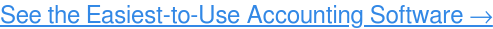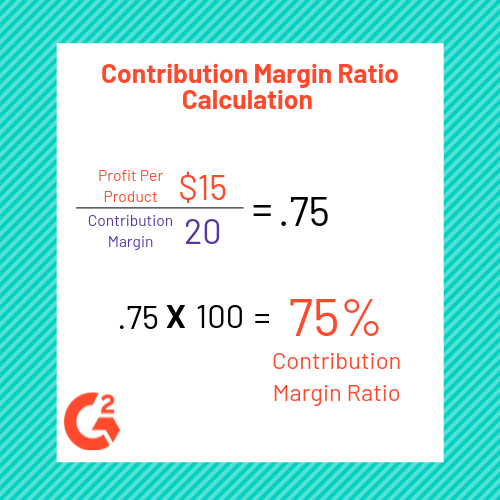As a business owner, you know how much goes into making your products.

From materials to labor to rent for your building, there are ample costs associated with the output of your company. Just looking at your balance sheet can tell you as much. And these costs all weigh heavily on your profits.

Contribution margin ratio is a useful metric that can take all of these costs into consideration on both a company-wide level and for the evaluation of one particular unit. Contribution margin represents the total amount of assets available to pay for fixed expenses while still  generating a profit. Finding the contribution margin ratio yields the proportion of profit generated on a sale.

Essentially, the contribution margin ratio reveals the percentage of each dollar that can cover a company’s fixed expenses. Let’s dive deeper into what goes into calculating it and why it is important for your business.

# What is contribution margin ratio?

Calculating contribution margin ratio can provide insight and clarity into how the operations of your business are affecting your total revenue. Along with other key accounting terms, contribution margin ratio can provide a clear picture of your company’s financial health. Using accounting software can also be an immense help in measuring your expenses and profits in operating a business.## Key elements of contribution margin ratio

Contribution margin ratio takes every cost associated with your company’s production into account in yielding the dollar contribution per unit of your products. In doing this, there are two types of expenses that you must recognize in calculating contribution margin ratio.

The first of these types of expenses are fixed expenses. These expenses do not typically depend on changes in the quantity of products your company makes. Fixed expenses include the rent for your building, property taxes, and insurance costs. Regardless of whether your company produces millions of material products or sells intangible products such as software, these expenses remain consistent.

The other type of expenses are variable expenses. Variable expenses directly depend upon the quantity of products produced by your company. These expenses include materials, labor, packaging, and equipment.

## How to calculate contribution margin

After identifying these expenses, it’s time to find the contribution margin. The contribution margin is calculated by finding the variable cost per unit. Divide the total amount of your variable costs by the number of units produced. Then, subtract the variable cost per unit from the cost per unit. That figure is the contribution margin.

Now here comes the important part. To calculate contribution margin ratio, divide the contribution margin you just found by the sales income of an individual product. This equation yields your contribution margin ratio, which gives you the percentage each product sale contributes toward the total fixed expenses that you need to cover each month.In the example above, the equation results in a contribution margin ratio of 75 percent (15/20). What does this mean? 75% of each product sale is available to contribute towards the total amount of fixed expenses that this business needs to cover each month to achieve the target monthly profit.

## Why contribution margin ratio is important

Contribution margin ratio provides insight into how your company’s products and sales fit into the bigger picture of your business. If the contribution margin ratio for a particular product is low or negative, it can be a sign that the product is not helping your company make a profit and should be sold at a different price point or not at all. It is also a helpful metric to track over time when looking at how sales affect profits.

### Get calculating

Now that we’ve overviewed the basics of contribution margin ratio, it’s time to get calculating on your own. Using the aforementioned formula, find your contribution margin and then divide it by the sales income of an individual product to yield your contribution margin ratio.

Never miss a post.

Subscribe to keep your fingers on the tech pulse.